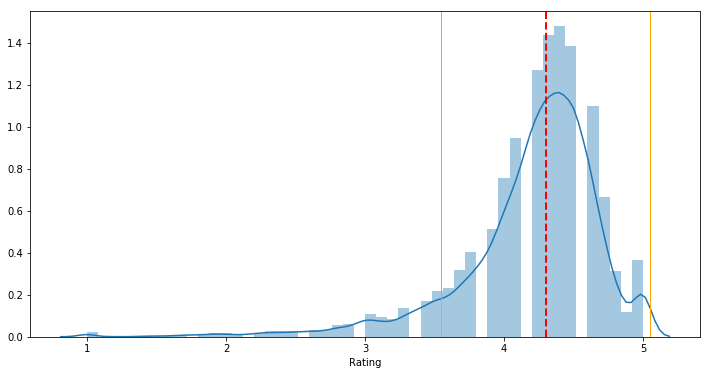# Estimates of Dispersion

Last updated: January 13th, 2020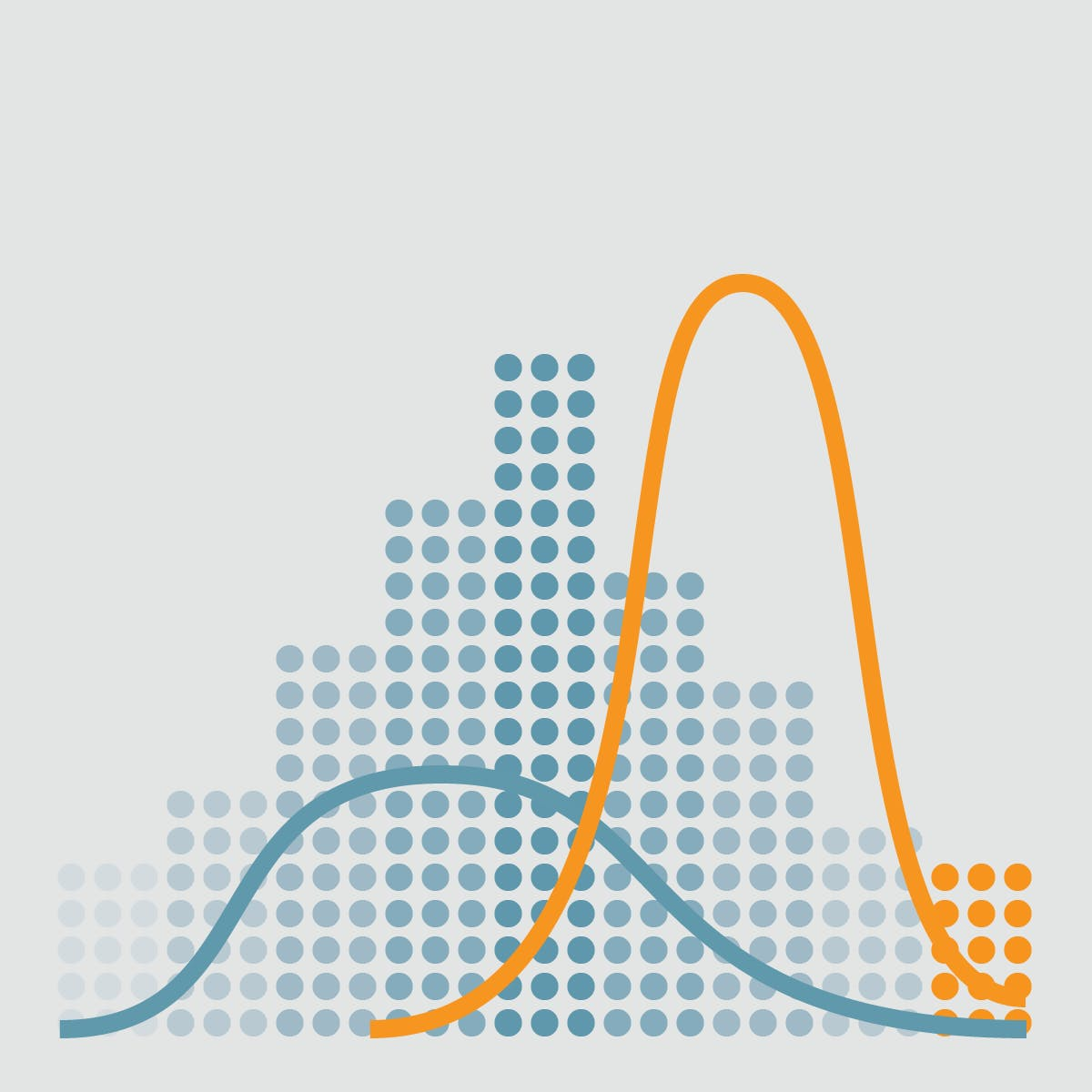# Estimates of Dispersion¶

Location is just one dimension in summarizing a feature. A second dimension, dispersion, also referred to as variability, measures whether the data values are tightly clustered or spread out.## Hands on!¶

In :
import numpy as np
import pandas as pd
import matplotlib.pyplot as plt
import seaborn as sns

%matplotlib inline## Undestanding what dispersion is¶

In statistics, dispersion (also called variability, scatter, or spread) is the extent to which a distribution is stretched or squeezed. Common examples of measures of statistical dispersion are the variance, standard deviation, and interquartile range.

Dispersion is contrasted with location or central tendency, and together they are the most used properties of distributions.

In :
plt.figure(figsize=(14,6))

data_1 = np.random.normal(0, 1, 100)
data_2 = np.random.normal(0, 2, 100)
data_3 = np.random.normal(0, 3, 100)

sns.distplot(data_1, hist=False)
sns.distplot(data_2, hist=False)
sns.distplot(data_3, hist=False)

plt.axvline(0, color='r', linestyle=':') # mean

Out:
<matplotlib.lines.Line2D at 0x7fbf594096a0>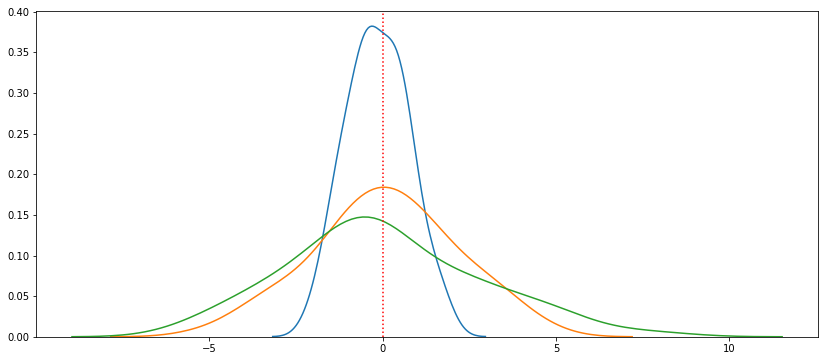## Cake slices example¶

Now let's say we have chocolate and cheese slices of cakes. Each slice will have different weights, take a look at the following table:Chocolate slices Cheese slices
100.00g 100.20g
100.02g 99.80g
99.97g 100.00g
100.03g 99.50g
99.98g 100.50g
In :
chocolate_slices = np.array([100.00, 100.02, 99.97, 100.03, 99.98])

cheese_slices = np.array([100.20, 99.80, 100.00, 99.50, 100.50])


Both types of slices have the same average (mean) weight:

In :
chocolate_slices.mean()

Out:
100.0
In :
cheese_slices.mean()

Out:
100.0

But take a look at the weights per type:

• Chocolate slices are almost equally weighted, so its distribution has less dispersion.
• Cheese slices has more difference between its weights, so its distribution has more dispersion.
In :
fig, (ax1, ax2) = plt.subplots(2, 1, sharex=True, figsize=(14,8))

sns.distplot(chocolate_slices, hist=False, rug=True, rug_kws={'color': 'r', 'linewidth': 3}, ax=ax1)
ax1.axvline(chocolate_slices.mean(), linestyle=':') # mean

sns.distplot(cheese_slices, hist=False, rug=True, rug_kws={'color': 'r', 'linewidth': 3}, ax=ax2)
ax2.axvline(cheese_slices.mean(), linestyle=':') # mean

ax1.title.set_text('Chocolate slices')
ax1.axes.get_yaxis().set_visible(False)

ax2.title.set_text('Cheese slices')
ax2.axes.get_yaxis().set_visible(False)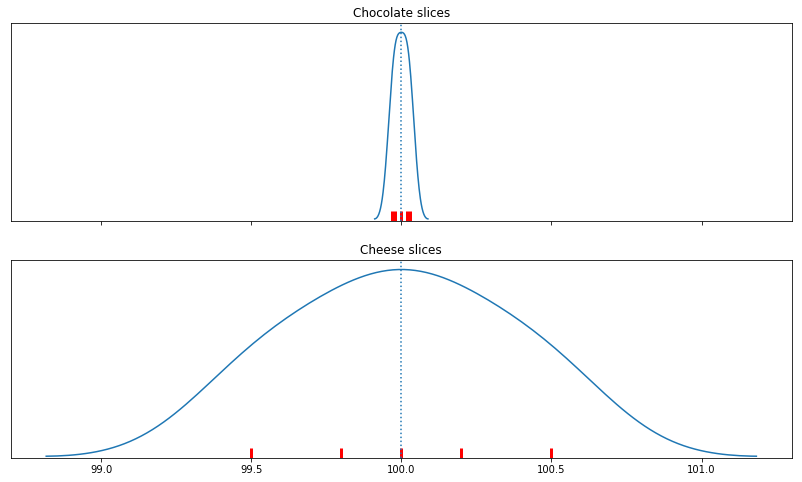## Variance and Standard Deviation¶

The best-known estimates for variability are the variance and the standard deviation, which are based on squared deviations between the estimate of location and the observed data.

### Variance¶

The variance is an average of the squared deviations:

$$Variance = s^2 = \frac{\sum\limits_{i}^n (x_i - \overline{x})^2}{n-1}$$

The square of the formula will make all the $(x_i - \overline{x})^2$ terms positive, and will penalize values furthest from the mean.

Supposing we have $mean = 100$:

• $x_1 = 101$ → $(101 - 100)^2$ = $1^2$ = 1
• $x_2 = 105$ → $(105 - 100)^2$ = $5^2$ = 25

We can easily calculate it in pandas with the var() method:

In :
chocolate_slices.var()

Out:
0.0005199999999999636
In :
cheese_slices.var()

Out:
0.11600000000000046

### Standard deviation¶

The standard deviation is the square root of the variance, and is much easier to interpret than the variance since it is on the same scale as the original data.

$$Standard \ deviation = s = \sigma = \sqrt{Variance}$$

Interestingly, standard deviation cannot be negative. A standard deviation close to 0 indicates that the data points tend to be close to the mean. The further the data points are from the mean, the greater the standard deviation.

Now we'll use the following Google Play Store Apps dataset and calculate both Variance and Standard deviation for its Rating column.

We can calculate the Standard Deviation as the square root of the variance, or directly (and preferably) with the std() method:

In :
df = pd.read_csv('data/googleplaystore.csv')


Out:
App Category Rating Reviews Installs Price Content Rating Genres Android Ver
0 Photo Editor & Candy Camera & Grid & ScrapBook ART_AND_DESIGN 4.1 159 10,000+ 0 Everyone Art & Design 4.0.3 and up
1 Coloring book moana ART_AND_DESIGN 3.9 967 500,000+ 0 Everyone Art & Design;Pretend Play 4.0.3 and up
2 U Launcher Lite – FREE Live Cool Themes, Hide ... ART_AND_DESIGN 4.7 87510 5,000,000+ 0 Everyone Art & Design 4.0.3 and up
3 Sketch - Draw & Paint ART_AND_DESIGN 4.5 215644 50,000,000+ 0 Teen Art & Design 4.2 and up
4 Pixel Draw - Number Art Coloring Book ART_AND_DESIGN 4.3 967 100,000+ 0 Everyone Art & Design;Creativity 4.4 and up
In :
df['Rating'].var()

Out:
0.2654504722754168
In :
import math

math.sqrt(df['Rating'].var())

Out:
0.5152188586177886
In :
df['Rating'].std()

Out:
0.5152188586177886

Both variance and standar deviation are especially sensitive to outliers since they are based on the squared deviations.

In :
plt.figure(figsize=(12,6))

sns.distplot(df['Rating'].dropna())

# Mean line
plt.axvline(df['Rating'].mean(), color='r', linestyle='dashed', linewidth=2)

# Standard deviation lines
plt.axvline(df['Rating'].mean() + df['Rating'].std(), linestyle='dotted', linewidth=2)
plt.axvline(df['Rating'].mean() - df['Rating'].std(), linestyle='dotted', linewidth=2)

Out:
<matplotlib.lines.Line2D at 0x7fbf56979518>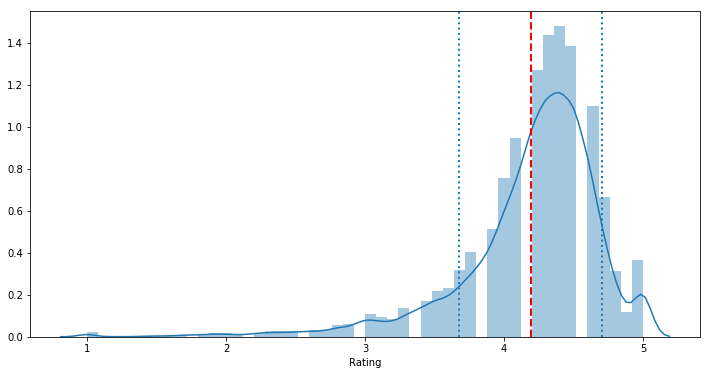In :
plt.figure(figsize=(12,6))

sns.distplot(df['Rating'].dropna())

# Mean line
plt.axvline(df['Rating'].mean(), color='#e74c3c', linestyle='dashed', linewidth=2)

# Standard deviation lines
plt.axvline(df['Rating'].mean() + df['Rating'].std(), linestyle='dotted', linewidth=2)
plt.axvline(df['Rating'].mean() - df['Rating'].std(), linestyle='dotted', linewidth=2)

plt.axvline(df['Rating'].mean() + 2 * df['Rating'].std(), color='g', linestyle='dotted', linewidth=2)
plt.axvline(df['Rating'].mean() - 2 * df['Rating'].std(), color='g', linestyle='dotted', linewidth=2)

Out:
<matplotlib.lines.Line2D at 0x7fbf568dc400>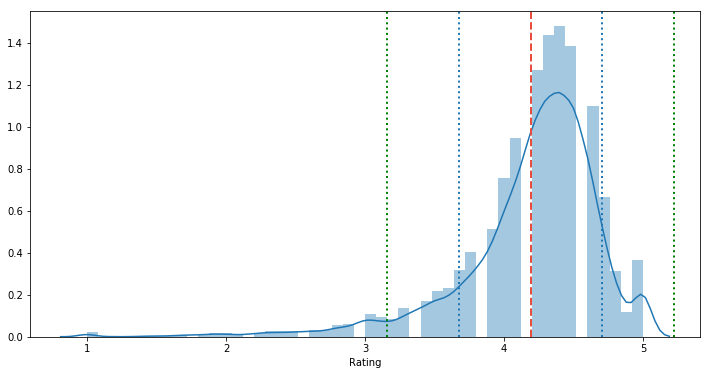## Estimates based on percentiles¶

Any set of data, arranged in ascending or descending order, can be divided into various parts, also known as partitions or subsets, regulated by quantiles. Quantile is a generic term for those values that divide the set into partitions of size n, so that each part represents 1/n of the set. Quantiles are not the partition itself, they are the numbers that define the partition. You can think of them as a sort of numeric boundary.

Before we saw how to get the median of the data by getting the value at the exact 50% of the data:

$$6, 2, 9, 3, 13, 4, 9, 7, 12, 8, 10, 5, 13, 9, 3$$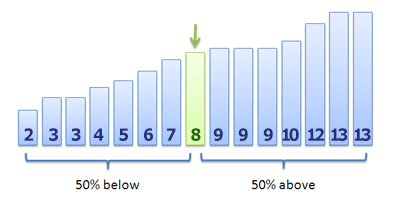In :
numbers = [6,2,9,3,13,4,9,7,12,8,10,5,13,9,3]

np.median(numbers)

Out:
8.0

Now we'll explore other possibilities by dividing the data using percentiles and quartiles:

### Percentiles¶

A percentile is a measure at which that percentage of the total values are the same as or below that measure. For example, 90% of the data values lie below the 90th percentile, whereas 10% of the data values lie below the 10th percentile.

$$percentile(n) = \frac{number\ of\ values\ below\ n}{size\ of\ set\ x} * 100$$

### Quartiles¶

Quartiles are values that divide a (part of a) data table into four groups containing an approximately equal number of observations. The total of 100% is split into four equal parts: 25%, 50%, 75% and 100%.

The first quartile (or lower quartile), Q1, is defined as the value that has an f-value equal to 0.25. This is the same thing as the twenty-fifth percentile.

The second quartile always corresponds to the median of the set x.

The third quartile (or upper quartile), Q3, has an f-value equal to 0.75.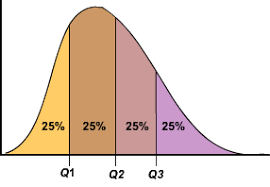In :
df['Rating'].mean()

Out:
4.191757420456972
In :
df['Rating'].median()

Out:
4.3
In :
df['Rating'].quantile(0.5)

Out:
4.3
In :
quartiles = df['Rating'].quantile([0.25, 0.5, 0.75, 1])

quartiles

Out:
0.25    4.0
0.50    4.3
0.75    4.5
1.00    5.0
Name: Rating, dtype: float64
In :
plt.figure(figsize=(12,6))

sns.distplot(df['Rating'].dropna())

# Median line
plt.axvline(df['Rating'].median(), color='#e74c3c', linestyle='dashed', linewidth=2)

for i, q in enumerate(quartiles):
# Quartile i line
plt.axvline(q, color='g', linestyle='dotted', linewidth=2)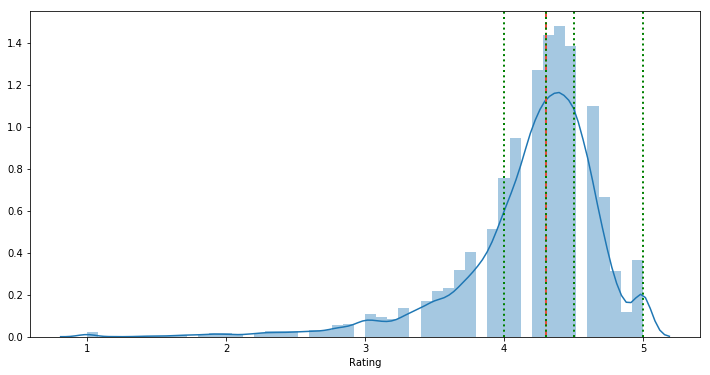In :
percentiles = df['Rating'].quantile(np.arange(0.1, 1.1, 0.1))

percentiles

Out:
0.1    3.6
0.2    3.9
0.3    4.1
0.4    4.2
0.5    4.3
0.6    4.4
0.7    4.5
0.8    4.6
0.9    4.7
1.0    5.0
Name: Rating, dtype: float64
In :
plt.figure(figsize=(12,6))

sns.distplot(df['Rating'].dropna())

# Median line
plt.axvline(df['Rating'].median(), color='r', linestyle='dashed', linewidth=2)

for i, q in enumerate(percentiles):
# Percentile i line
plt.axvline(q, color='g', linestyle='dotted', linewidth=2)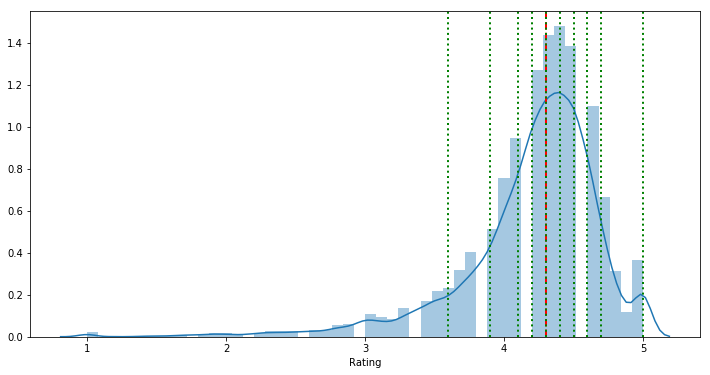### Interquartile range (IQR)¶

Interquartile range (IQR) measure the "spread" in a data set. Looking at spread lets us see how much data varies. Range is a quick way to get an idea of spread. It takes longer to find the IQR, but it sometimes gives us more useful information about spread.

The IQR describes the middle 50% of values when ordered from lowest to highest.

To find the interquartile range (IQR), first find the median (middle value) of the lower and upper half of the data. In other words, it is the distance between the first quartile ${Q}_1$ and the third quartile ${Q}_3$.

$${IQR}= {Q}_3 - {Q}_1$$
In :
quartiles = df['Rating'].quantile([0.25, 0.5, 0.75, 1])

iqr = quartiles[0.75] - quartiles[0.25]

iqr

Out:
0.5
In :
df['Rating'].std()

Out:
0.5152188586177886
In :
plt.figure(figsize=(12,6))

sns.distplot(df['Rating'].dropna())

# Median line
plt.axvline(df['Rating'].median(), color='r', linestyle='dashed', linewidth=2)

# 1.5 * IQR line
plt.axvline(df['Rating'].median() - (1.5 * iqr), color='orange', linestyle='-', linewidth=1)
plt.axvline(df['Rating'].median() + (1.5 * iqr), color='orange', linestyle='-', linewidth=1)

Out:
<matplotlib.lines.Line2D at 0x7fbf55f4e978>